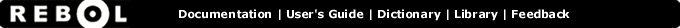# Cosine - Function Summary

## Summary:

Returns the trigonometric cosine in degrees.

cosine value

## Arguments:

value - The value argument. (must be: number)

## Refinements:

/radians - Value is specified in radians.

## Description:

Ratio between the length of the adjacent side to the length of the hypotenuse of a right triangle.

 ``` print cosine 90 0.0```

 ``` print (cosine 45) = (sine 45) true```

 ``` print cosine/radians pi -1.0```

## Related:

arccosine - Returns the trigonometric arccosine in degrees.
arcsine - Returns the trigonometric arcsine in degrees.
arctangent - Returns the trigonometric arctangent in degrees.
sine - Returns the trigonometric sine in degrees.
tangent - Returns the trigonometric tangent in degrees.